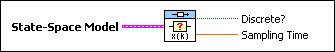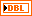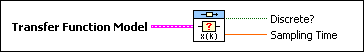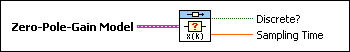# CD Verify if Discrete VI

LabVIEW 2018 Control Design and Simulation Module Help

Edition Date: March 2018

Part Number: 371894J-01

»View Product InfoDownload Help (Windows Only)

Owning Palette: Model Information VIs

Requires: Control Design and Simulation Module

Checks if the input model represents a discrete-time system. Wire data to the State-Space Model input to determine the polymorphic instance to use or manually select the instance.

Use the pull-down menu to select an instance of this VI.

 Select an instance CD Verify if Discrete (State-Space)CD Verify if Discrete (Transfer Function)CD Verify if Discrete (Zero-Pole-Gain)

## CD Verify if Discrete (State-Space)State-Space Model contains a mathematical representation of and information about a system that this VI checks to determine if it represents a discrete-time system.If Discrete? is TRUE, the model represents a discrete-time system and the Sampling Time of the model is greater than zero.Sampling Time returns the sampling time of the model. Sampling Time defines whether the model represents a continuous-time system or a discrete-time system. If the model represents a continuous-time system, Sampling Time equals zero. If the model represents a discrete-time system, Sampling Time is greater than zero and equal to the sampling rate, in seconds, of the discrete system.

## CD Verify if Discrete (Transfer Function)Transfer Function Model contains a mathematical representation of and information about the system that this VI checks to determine if it represents a discrete-time system.If Discrete? is TRUE, the model represents a discrete-time system and the Sampling Time of the model is greater than zero.Sampling Time returns the sampling time of the model. Sampling Time defines whether the model represents a continuous-time system or a discrete-time system. If the model represents a continuous-time system, Sampling Time equals zero. If the model represents a discrete-time system, Sampling Time is greater than zero and equal to the sampling rate, in seconds, of the discrete system.

## CD Verify if Discrete (Zero-Pole-Gain)Zero-Pole-Gain Model contains a mathematical representation of and information about a system that this VI checks to determine if it represents a discrete-time system.If Discrete? is TRUE, the model represents a discrete-time system and the Sampling Time of the model is greater than zero.Sampling Time returns the sampling time of the model. Sampling Time defines whether the model represents a continuous-time system or a discrete-time system. If the model represents a continuous-time system, Sampling Time equals zero. If the model represents a discrete-time system, Sampling Time is greater than zero and equal to the sampling rate, in seconds, of the discrete system.